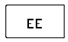## Scientific Notation key Assignment Help

Assignment Help: >> Scientific Notation - Scientific Notation key

Scientific Notation key:If pressed after a number is entered on the display then the EE key will convert the number into scientific notation. Pressing the EE key tells the calculator the further entered numbers are the exponential values if a number is to be entered in scientific notation within the calculator,.

Scientists, engineers, operators & technicians use scientific notation while working with extremely large and extremely small numbers.  A speed of light is 29,900,000,000 centimeters per second and the mass of an electron is 0.000549 atomic mass units.  It is simpler to express these numbers in a shorter way known as scientific notation, therefore preventing the writing of several zeros and transposition errors.

29,900,000,000 = 2.99 x 1010

0.000549 = 5.49 x 10-4